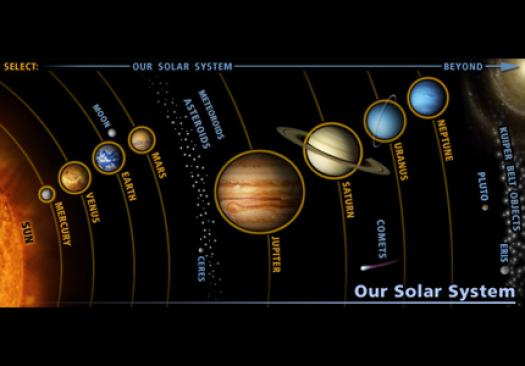# 4th Quarter Practice Assessment

55 Questions | Attempts: 647SettingsTaking this practice assessment is a great way to study for the assessment and see what you have learned and what you still need to review.

• 1.
About how many galaxies are there?
• A.

Less than 100

• B.

Hundreds

• C.

Thousands

• D.

Millions

• E.

Billions

• 2.
Galaxies that have no shape are called _________________ galaxies.
• A.

Spiral

• B.

Irregular

• C.

Elliptical

• D.

Spherical

• E.

Twirl

• 3.
Galaxies that look like a flattened ball are called _________________ galaxies.
• A.

Spiral

• B.

Irregular

• C.

Elliptical

• D.

Spherical

• E.

Twirl

• 4.
Galaxies shaped like a pinwheel are called _________________ galaxies.
• A.

Spiral

• B.

Irregular

• C.

Elliptical

• D.

Spherical

• E.

Twirl

• 5.
Our galaxy is:
• A.

A twirl galaxy called the swirl galaxy

• B.

A spiral galaxy called the milky way galaxy

• C.

An elliptical galaxy called the milky way galaxy

• D.

An elliptical galaxy called the swirl galaxy

• E.

A spiral galaxy called the swirl galaxy

• 6.
Put the items in order from largest to smallest.
• A.

Universe, solar system, galaxy

• B.

Solar system, universe, galaxy

• C.

Universe, galaxy, solar system

• D.

Galaxy, universe, solar system

• E.

Galaxy, solar system, universe

• 7.
At the center of our solar system is
• A.

The moon

• B.

Earth

• C.

A galaxy

• D.

A black hole

• E.

A star

• 8.
How many stars are there in our solar system
• A.

One

• B.

Around ten

• C.

Thousands

• D.

Millions

• E.

Billions

• 9.
The inner-most layer of the sun, where all of its energy is produced is the
• A.

Photosphere

• B.

• C.

Corona

• D.

Core

• E.

Convective zone

• 10.
About how many times larger is Jupiter than Neptune?  Neptune's diameter is 49,528 and Jupiter's diameter is 142,984.
• A.

1

• B.

2

• C.

3

• D.

4

• E.

5

• 11.
The layer of the sun we can only see during a solar eclipse is called the
• A.

Core

• B.

Corona

• C.

Convective zone

• D.

Solar flare

• E.

Photosphere

• 12.
Cool, dark spots on the sun's surface are called
• A.

Prominences

• B.

Solar flares

• C.

Sun spots

• D.

Coronal mass ejections

• E.

Pleges

• 13.
One rotation is equal to
• A.

One hour

• B.

One day

• C.

One month

• D.

One year

• E.

• 14.
One revolution is equal to
• A.

One hour

• B.

One day

• C.

One month

• D.

One year

• E.

• 15.
This picture is of a(n)
• A.

Lunar eclipse

• B.

Solar flare

• C.

Solar eclipse

• D.

Annular eclipse

• 16.
The movement of one celestial object around another is called
• A.

A trip

• B.

A revolution

• C.

A rotation

• D.

An orbit

• E.

A spin

• 17.
The spinning of a planet on its axis is called
• A.

Twirl

• B.

Ellipse

• C.

Revolution

• D.

Orbit

• E.

Rotation

• 18.
Are inner planets warmer than outer planets?
• A.

Yes

• B.

No

• 19.
Do inner planets have a faster rotation than outer planets?
• A.

Yes

• B.

No

• C.

Some do and some don't

• D.

No one knows

• 20.
Do inner planets have a faster revolution than the outer planets?
• A.

Yes

• B.

No

• C.

Some do and some don't

• D.

No one knows

• 21.
Are inner planets smaller or larger than the outer planets?
• A.

Smaller

• B.

Larger

• C.

Some are smaller and some are larger

• D.

No one knows

• 22.
Do the inner planets have a gassy or rocky surface?
• A.

Gassy

• B.

Rocky

• C.

Some are gassy and some are rocky

• D.

No one knows

• 23.
Diameter tells you about a sphere's
• A.

Color

• B.

Mass

• C.

Weight

• D.

Size

• 24.
Asteroids orbit between
• A.

The sun and Mercury

• B.

Earth and Mars

• C.

Mars and Jupiter

• D.

Jupiter and Saturn

• E.

Saturn and Uranus

• 25.
Meteoroids are _____________ than asteroids
• A.

Heavier

• B.

Rounder

• C.

Smaller

• D.

Larger

• E.

More metallic

## Related TopicsBack to top
×

Wait!
Here's an interesting quiz for you.